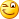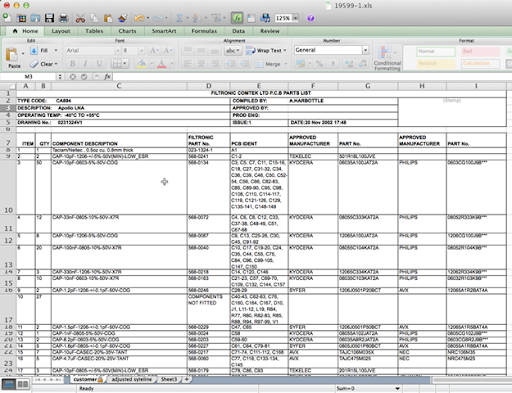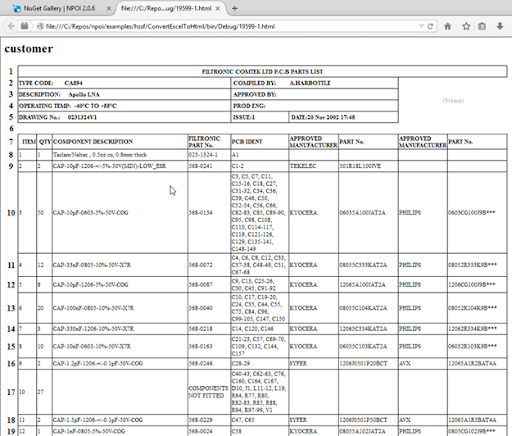## Pages

### NPOI 2.0 - Converting Excel XLS documents to HTML format

This is the 4th post of a series of posts about NPOI 2.0.

This time we’re gonna see how easy it is to grab a .XLS file and then have it converted to HTML code.

Note: in NPOI 2.0.6 only XLS format is supported. XLSX support is coming in a future release (maybe 2.1) because it still needs more testing.

Enough said, let’s get to the code:

```using NPOI.HSSF.Converter;
using NPOI.HSSF.UserModel;
using System;
using System.IO;

namespace NPOI.Examples.ConvertExcelToHtml
{
class Program
{
static void Main(string[] args)
{
HSSFWorkbook workbook;
// Excel file to convert
string fileName = "19599-1.xls";
fileName = Path.Combine(Environment.CurrentDirectory, fileName);

ExcelToHtmlConverter excelToHtmlConverter = new ExcelToHtmlConverter();

// Set output parameters
excelToHtmlConverter.OutputHiddenColumns = true;
excelToHtmlConverter.OutputHiddenRows = true;
excelToHtmlConverter.OutputRowNumbers = true;
excelToHtmlConverter.UseDivsToSpan = true;

// Process the Excel file
excelToHtmlConverter.ProcessWorkbook(workbook);

// Output the HTML file
excelToHtmlConverter.Document.Save(Path.ChangeExtension(fileName, "html"));
}
}
}```

The code above was taken from ConvertExcelToHtml sample project.

Here’s the sample spreadsheet open in Excel for Mac that was used as input:Figure 1 - Excel spreadsheet to be converted to HTML code

This is the HTML generated:Figure 2 - HTML output code generated from the conversion

Note that I used a screenshot above but it depicts the .html file (check the browser’s address bar).

Enjoy and have fun!